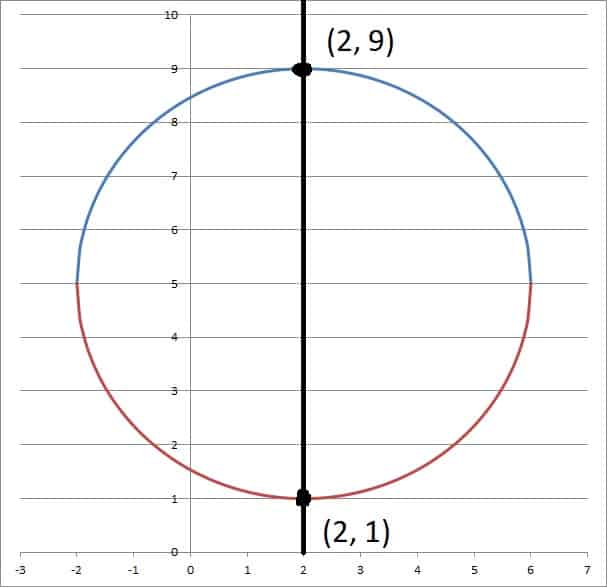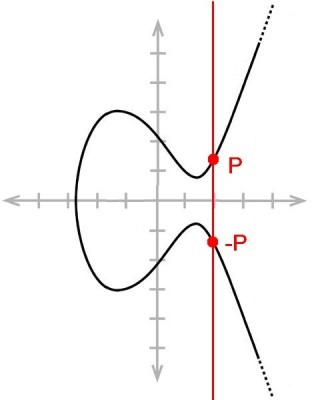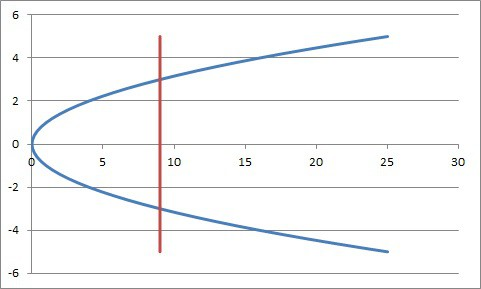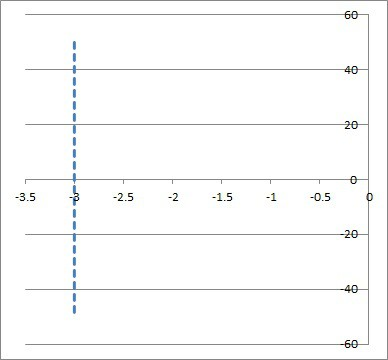# What Cannot Be A Function? (5 Key Examples To Know)

There are lots of graphs that are not functions.  These relations fail the vertical line test, meaning that there is some ambiguity in the output for a given input.

So, what cannot be a function?  Some graphs that cannot be functions include ellipses, elliptic curves, rectangles, sideways parabolas, and vertical lines. Each of these graphs fails the vertical line test, which tells us that there is at least one input (x-value) with two or more outputs (y-values).

Of course, there are other graphs that cannot be functions, but these five are a good start if you want to get a better sense of what is not a function.

In this article, we’ll take a closer look at each of these 5 non-functions and their graphs.  We’ll also talk a bit about the vertical line test and how to apply it in each case.

Let’s get started.

## What Cannot Be A Function?

There are lots of examples of graphs that cannot be functions.  Here are five examples of graphs cannot be functions (since they fail the vertical line test):

• Ellipses (a special case is a circle)
• Elliptic Curves (used in some types of cryptography)
• Rectangles (a special case is a square)
• Sideways Parabolas (graph of a quadratic)
• Vertical Lines

Let’s take a closer look at each of these relations in turn.

### Ellipses (Includes Circles)

An ellipse is a closed curve around two focal points (foci).  For all points on an ellipse, the sum of the distances to each focus is constant.

The equation of an ellipse is:

• (x – h)2/a2 + (y – k)2/b2 = 1 (assuming a > b)

where (h, k) is the center of the ellipse, a is the length of the semi-major axis, and b is the length of the semi-minor axis.  The foci are (h + c, k) and (h – c, k), where c = √(a2 – b2).

If a = b, then we have the special case of a circle with radius a (since a circle is a special case of an ellipse).  Note that a = b means c = 0, which tells us that the two foci of an ellipse converge into one to give us a circle with one focus (the center).

We can see from the ellipse equation that there is at least one vertical line that intersects the curve twice: the line x = h.  When we substitute x = h into the ellipse equation, we get:

• (x – h)2/a2 + (y – k)2/b2 = 1
• (h – h)2/a2 + (y – k)2/b2 = 1
• (0)2/a2 + (y – k)2/b2 = 1
• (y – k)2/b2 = 1
• (y – k)2 = b2
• y – k = +/- b
• y = k +/- b

So, the corresponding values of y are k + b and k – b.  So, both of the points (h, k + b) and (h, k – b) are both on the same vertical line (x = h) and on the ellipse.

This means that an ellipse fails the vertical line test, so it is not a function.  We can see this illustrated in the graph below.This circle (a special case of an ellipse) fails the vertical line test and is not a function, since the line x = 2 intersects the curve twice, at (2, 9) and (2, 1).

***Note: you can take part of a circle to get a function (or a one-to-one function). You can learn more in my article here.

### Elliptic Curves

An elliptic curve is a curve with the equation

• y2 = x3 + ax + b [assume 4a3 is not equal to 27b2]

where a and b are coefficients in the field over which the elliptic curve is defined.  Here, we will consider a and b to be real numbers.

Elliptic curves are used in cryptography (elliptic curve cryptography), which is used for data security and digital signatures (such as those used in the blockchain for Bitcoin).

An elliptic curve is not an ellipse, and it is also not a function.  For example, consider the values y = 1 and y = -1 in an elliptic curve equation.

For y = 1, we get:

• y2 = x3 + ax + b
• 12 = x3 + ax + b
• 1 = x3 + ax + b
• 0 = x3 + ax + (b – 1)

For y = -1, we get:

• y2 = x3 + ax + b
• (-1)2 = x3 + ax + b
• 1 = x3 + ax + b
• 0 = x3 + ax + (b – 1)

So for both y = 1 and y = -1, we get the same cubic equation: x3 + ax + (b – 1) = 0.  Since polynomials have complex conjugate roots, we know that this cubic equation has at least one real solution (there are either one or three real solutions).

Call this real solution r.  Then the points (r, 1) and (r, -1) are both on the elliptic curve.  Both of these points are on the vertical line x = r and on the elliptic curve.

This means that the elliptic curve fails the vertical line test, so it is not a function.  We can see this illustrated in the graph below.An elliptic curve fails the vertical line test and is not a function. For any point P = (x, y) with y not zero, there is a corresponding point -P = (x, -y) on the curve. The point -P is just the point P reflected across the x-axis (the horizontal line y = 0).

***Note: an elliptic curve is not the same as an ellipse, even though the names sound like they might be similar.

### Rectangles (Includes Squares)

A rectangle is another example of a relation that is not a function.  A square is a specific type of rectangle (where all sides are of equal length), so a square is also not a function.

This is fairly easy to see.  Consider the left side of a rectangle, which is a line segment of the form:

• x = a, for some values of y, such as c <= y <= d

Both of the points (a, c) and (a, d) are on the vertical line x = a, and both are on the perimeter of the rectangle.

This means that the rectangle fails the vertical line test, so it is not a function.  We can see this illustrated in the graph below.The left side of a rectangle is a vertical line segment. The top left and bottom left corners of the rectangle lie on the same vertical line and on the rectangle, so the rectangle fails the vertical line test and is not a function.

### Sideways Parabolas

A parabola that is “sideways” also cannot be a function.  This is a parabola of the form

• x = ay2 + by + c  [where a, b, and c are real and a is not zero]

It is fairly easy to see that a sideways parabola of this form is not a function.  Consider the value x = c in the parabola equation:

• x = ay2 + by + c
• c = ay2 + by + c
• 0 = ay2 + by
• 0 = y(ay + b)

This equation implies that y = 0 and y = -b/a are both solutions to the equation.  So, both of the points (c, 0) and (c, -b/a) are on the vertical line x = c and on the sideways parabola.

This means that the sideways parabola fails the vertical line test, so it is not a function.  We can see this illustrated in the graph below.This sideways parabola (blue curve) fails the vertical line test (since the red vertical line intersects the blue curve twice). Thus, it is not a function.

### Vertical Lines

A vertical line can never be a function, because it fails the vertical line test by definition.  A vertical line has the form

• x = a [where a is a real number]

Every point on x = a is on the line, including the points (a, 0) and (a, 1).  Since both of these points are on the same vertical line, we know that x = a fails the vertical line test.

Thus, x = a is not a function for any real value of a, as we can see in the graph below.The vertical line x = -3 fails the vertical line test, since the points (-3, 0) and (-3, 1) are both on the line. No vertical line is a function.

## Conclusion

Now you know about some graphs that are not functions.  You also know how to reason through why they are not functions (failing the vertical line test).